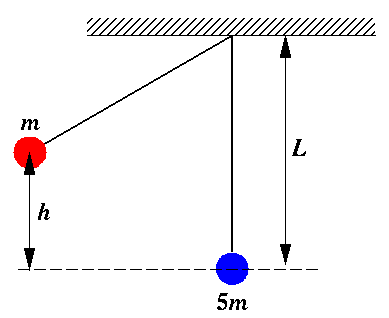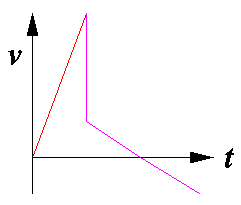Directions: Select the best possible answer from the choices A-D. Guessing will not (on average) reduce your score, but if you have no idea how to work a problem, leave it blank and I'll give you the expected ¼ point.

This is a timed test. Your start time is: 9:12

SHOW= (SHOW=4 is a good start)

## Ballistic PendulumA red sphere (of mass m) and a blue sphere (of mass 5m) are attached to the ceiling by massless strings of identical length forming twin pendulums of length L. The red sphere is drawn to the left so that its center of mass has been raised a distance h and is then released. As the red sphere moves back through equilibrium it hits and adheres to the blue sphere. The combined system swings to the right, eventually reaching some maximum height before returning to equilibrium.

1. How much work had to be done to move the red sphere from equilibrium to its release point (as displayed above).
1. mgh
2. -mgh
3. ½ mgh
4. cannot be determined
```A B C D
```

2. If the post-collision speed of the combined spheres is initially v, what maximum height will be achieved?
1. v2/(2gL)½
2. mgh
3. v2/g
4. v2/(2g)
```A B C D
```

3. If the collision were elastic rather than inelastic how would the post-elastic-collision speeds compare to the post-inelastic-collision speed?
1. The red sphere would be moving faster, the blue slower.
2. The red sphere would be moving slower, the blue faster.
3. Both red and blue would be moving faster than the post-inelastic-collision speed.
4. Neither red and blue would be moving faster than the post-inelastic-collision speed.
```A B C D
```

4. Which graph best represents the horizontal velocity of the red sphere as a function of time?

ABCD```A B C D
```

5. Aside from the moment of the collision, the acceleration of the red sphere is largest:
1. at the time of release
2. just before the collision
3. just after the collision
4. when the combined spheres reach their maximum height
```A B C D
```